# Affine Algebraic Sets - Dummit and Foote, page 660, Ex. 3

• I
• Math Amateur
This can be written as ##e_n \cdot x^n \cdot y^2 = (x^3-y^2) \cdot (-e_n x^n) + e_n \cdot x^{n+3}.##The first factor at ##(x^3-y^2)## will be used to build up ##g(x,y)## and the second will be added to ##f_0.##You can proceed this way with every following term ##c_n y^n f_n(x)

#### Math Amateur

Gold Member
MHB
I am trying to gain an understanding of the basics of elementary algebraic geometry and am reading Dummit and Foote Chapter 15: Commutative Rings and Algebraic Geometry ...

At present I am focused on Section 15.1 Noetherian Rings and Affine Algebraic Sets ... ...

I need someone to help me to fully understand the reasoning/analysis behind one of the statements in Example (3) on Page 660 of D&F ...

On page 660 (in Section 15.1) of D&F we find the following text and examples (I am specifically focused on Example (3)):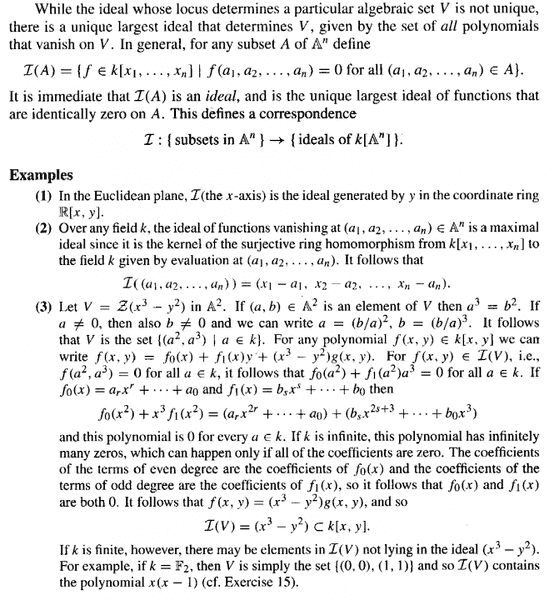In the above text, in Example (3), we find the following:

"... ... For any polynomial ##f(x,y) \in k[x,y]## we can write

##f(x,y) = f_0(x) + f_1(x)y + (x^3 - y^2) g(x,y).##"

Can someone explain (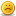slowly and carefully) exactly how/why this is true ... ...

Peter

====================================================

In order for readers of the above post to understand the context of the question and the notation employed I am providing the introductory pages on affine algebraic sets in the D&F text ... ... as follows: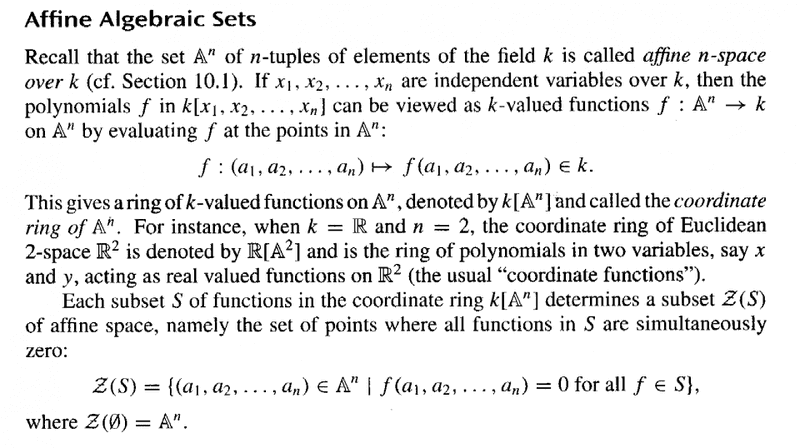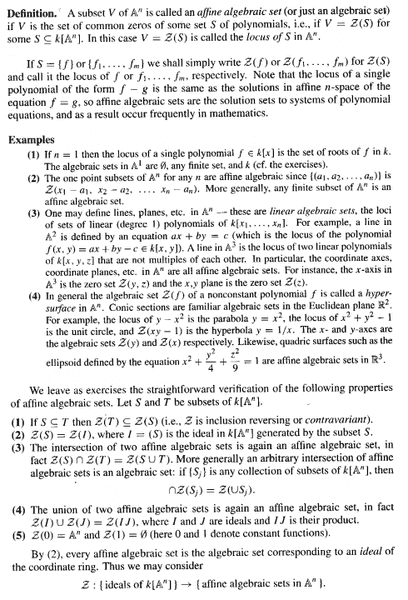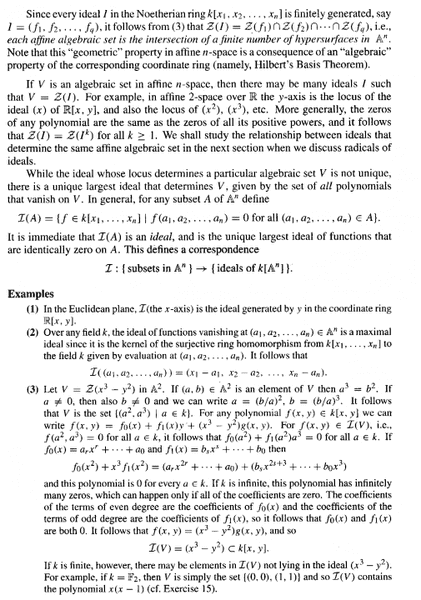#### Attachments

Math Amateur said:
I am trying to gain an understanding of the basics of elementary algebraic geometry and am reading Dummit and Foote Chapter 15: Commutative Rings and Algebraic Geometry ...

At present I am focused on Section 15.1 Noetherian Rings and Affine Algebraic Sets ... ...

I need someone to help me to fully understand the reasoning/analysis behind one of the statements in Example (3) on Page 660 of D&F ...

On page 660 (in Section 15.1) of D&F we find the following text and examples (I am specifically focused on Example (3)):In the above text, in Example (3), we find the following:

"... ... For any polynomial ##f(x,y) \in k[x,y]## we can write

##f(x,y) = f_0(x) + f_1(x)y + (x^3 - y^2) g(x,y).##"

Can someone explain (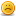slowly and carefully) exactly how/why this is true ... ...

Peter

Hi Peter,

let us start and consider ##f(x,y)## as polynomial in ##y##. Then it can be written:
##f(x,y) = c_0 \cdot f_0(x) + c_1 \cdot y \cdot f_1(x) + c_2 \cdot y^2 \cdot f_2(x) + \dots##
What we are doing next is to gather everything, that "disturbs" us and putting it into the terms ##f_0## and ## f_1##.
There are no restrictions on those, beside that they aren't allowed to contain ##y##'s. so our first substitution will be ##f_0^{new} = c_0 f_0## and ##f_1^{new} = c_1 f_1##. For the next steps I will drop the ##^{new}## because it is not necessary to keep a record on them.

Now ##c_2 \cdot y^2 \cdot f_2(x) = c_2 \cdot y^2 \cdot (d_0 + d_1 \cdot x + d_2 \cdot x^2 + \dots) = c_2d_0 y^2 + c_2d_1xy^2+c_2d_2x^2y^2 + \dots## and every term is of the form ##e_n \cdot x^n \cdot y^2.##
This can be written as ##e_n \cdot x^n \cdot y^2 = (x^3-y^2) \cdot (-e_n x^n) + e_n \cdot x^{n+3}.##
The first factor at ##(x^3-y^2)## will be used to build up ##g(x,y)## and the second will be added to ##f_0.##

You can proceed this way with every following term ##c_n y^n f_n(x) \; (n>2)##, i.e. perform the long division on polynomials.

•WWGD and Math Amateur
To summarize fresh34's nice answer, for any domain R, we can divide by any polynomial in the ring R[Y] whose lead coefficient is a unit. E.g. we can always divide by Y^2 + aY + b. Moreover, as is usual in long division, the remainder has lower degree than the divisor.

Thus if we take R = k[X], then in the ring R[Y] = k[X][Y] ≈ k[X,Y], we can divide any polynomial by Y^2 - X^3, and get a remainder of degree lower than 2 (in Y). I.e. the remainder will have form f1Y + f0 where f0 and f1 lie in the coefficient ring k[X].

The point of this somewhat tedious example is to illustrate that over a finite field, one does not keep much information about a polynomial from looking only where it vanishes, since over Z/2Z, any multiple of the polynomial x(x-1) vanishes everywhere. to insure that the zero locus has as much information as possible, one usually assumes the field algebraically closed. see e.g. hilbert's nullstellensatz in DF, p.675.

Last edited:
•Math Amateur
fresh_42 said:
Hi Peter,

let us start and consider ##f(x,y)## as polynomial in ##y##. Then it can be written:
##f(x,y) = c_0 \cdot f_0(x) + c_1 \cdot y \cdot f_1(x) + c_2 \cdot y^2 \cdot f_2(x) + \dots##
What we are doing next is to gather everything, that "disturbs" us and putting it into the terms ##f_0## and ## f_1##.
There are no restrictions on those, beside that they aren't allowed to contain ##y##'s. so our first substitution will be ##f_0^{new} = c_0 f_0## and ##f_1^{new} = c_1 f_1##. For the next steps I will drop the ##^{new}## because it is not necessary to keep a record on them.

Now ##c_2 \cdot y^2 \cdot f_2(x) = c_2 \cdot y^2 \cdot (d_0 + d_1 \cdot x + d_2 \cdot x^2 + \dots) = c_2d_0 y^2 + c_2d_1xy^2+c_2d_2x^2y^2 + \dots## and every term is of the form ##e_n \cdot x^n \cdot y^2.##
This can be written as ##e_n \cdot x^n \cdot y^2 = (x^3-y^2) \cdot (-e_n x^n) + e_n \cdot x^{n+3}.##
The first factor at ##(x^3-y^2)## will be used to build up ##g(x,y)## and the second will be added to ##f_0.##

You can proceed this way with every following term ##c_n y^n f_n(x) \; (n>2)##, i.e. perform the long division on polynomials.

Thanks fresh_42 ... that post was most helpful ...

You really helped me when you wrote:

"This can be written as ##e_n \cdot x^n \cdot y^2 = (x^3-y^2) \cdot (-e_n x^n) + e_n \cdot x^{n+3}.##
The first factor at ##(x^3-y^2)## will be used to build up ##g(x,y)## and the second will be added to ##f_0.##"

I was really perplexed at how to deal with this situation ...

Thanks again for the help,

Peter

mathwonk said:
To summarize fresh34's nice answer, for any domain R, we can divide by any polynomial in the ring R[Y] whose lead coefficient is a unit. E.g. we can always divide by Y^2 + aY + b. Moreover, as is usual in long division, the remainder has lower degree than the divisor.

Thus if we take R = k[X], then in the ring R[Y] = k[X][Y] ≈ k[X,Y], we can divide any polynomial by Y^2 - X^3, and get a remainder of degree lower than 2 (in Y). I.e. the remainder will have form f1Y + f0 where f0 and f1 lie in the coefficient ring k[X].

The point of this somewhat tedious example is to illustrate that over a finite field, one does not keep much information about a polynomial from looking only where it vanishes, since over Z/2Z, any multiple of the polynomial x(x-1) vanishes everywhere. to insure that the zero locus has as much information as possible, one usually assumes the field algebraically closed. see e.g. hilbert's nullstellensatz in DF, p.675.

Thanks mathwonk ... really helpful to get a general perspective on this matter ...

Thank you for moving my understanding forward ... really appreciate it ...

Peter

You're welcome. It's always a pleasure to read your carefully prepared and precise questions.

•Math Amateur